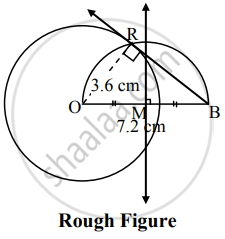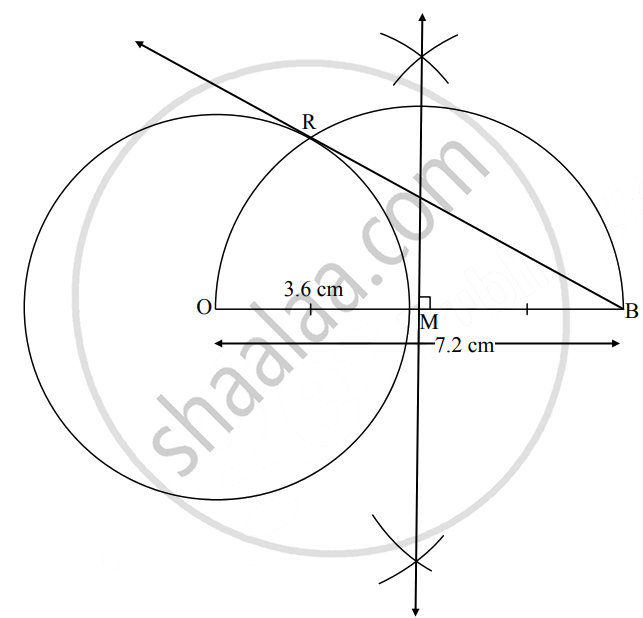# Draw a circle with center O and radius 3.6 cm. Draw a tangent to the circle from point B at a distance of 7.2 cm from the center of the circle. - Geometry

Diagram

Draw a circle with center O and radius 3.6 cm. Draw a tangent to the circle from point B at a distance of 7.2 cm from the center of the circle.

#### SolutionAnalysis:

As shown in the figure, let B be a point in the exterior of the circle at a distance of 7.2 cm from O.

Let BR be the tangent to the circle at points R.

∴ seg OR ⊥ tangent BR   ......[Tangent theorem]

∴ ∠ORB = 90°

∴ Point R is on the circle having OB as diameter.   ......[Angle inscribed in a semicircle is a right angle]

On drawing a circle with OB as diameter, the point where it intersects the circle with centre O, will be the position of point R.Steps of construction:

1. With centre O, draw a circle of radius 3.6 cm
2. Take point B such that OB = 7.2 cm
3. Draw the perpendicular bisector of seg OB. It intersects OB in point M.
4. With M as centre and radius equal to OM, draw an arc intersecting the circle in points R.
5. Draw ray BR.
Ray BR is the required tangent to the circle.
Concept: Construction of a Tangent to the Circle at a Point on the Circle
Is there an error in this question or solution?

Share